29 February 2016

1、单个模型：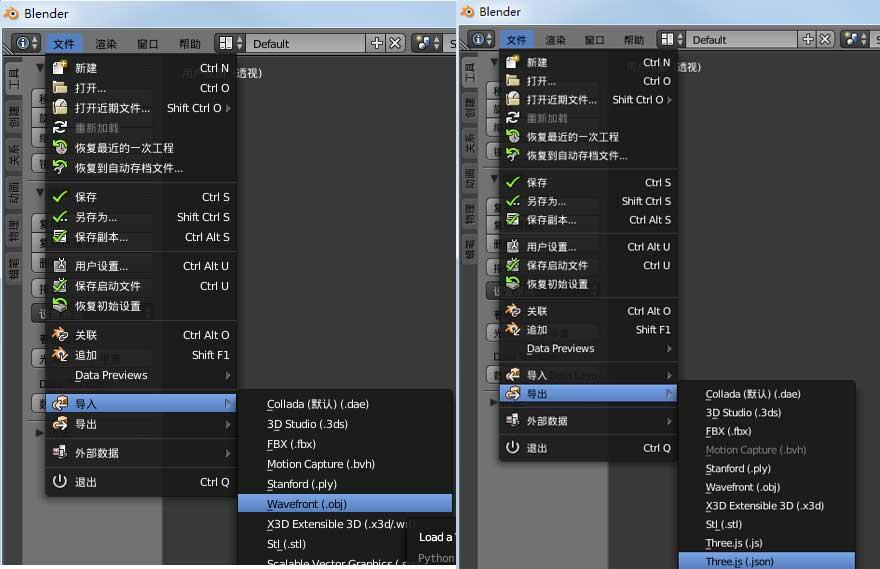图一 (左边为导入obj，右边导出json)

2、动画模型：

（1）选择好动画的帧数，如果没选择，导出的json文件会有空帧。并且文件也会相对增大。

（2）选择好导出选项中Animation，一般就选择Morph Animation、Embed Animation选项。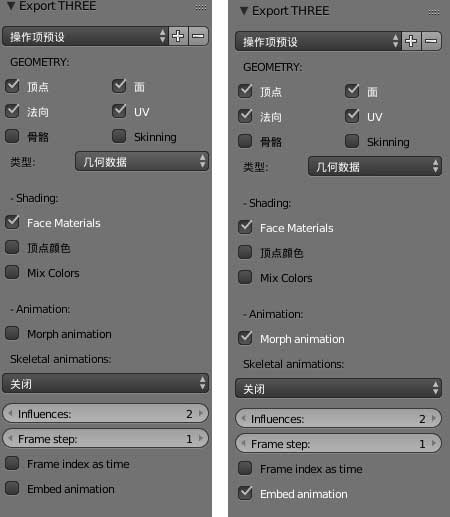图二 (左边为导出单个模型，右边导出动画模型)

（1）将模型的面和顶点在不影响正常显示的情况下进行删减

（2）对动画模型的帧数、面、顶点也进行删减

（1）模型材质丢失

（2）模型的大小太大（模型量少大小还可以接受，考虑到此次项目中的模型量多，估算了一下大概有70-80M左右）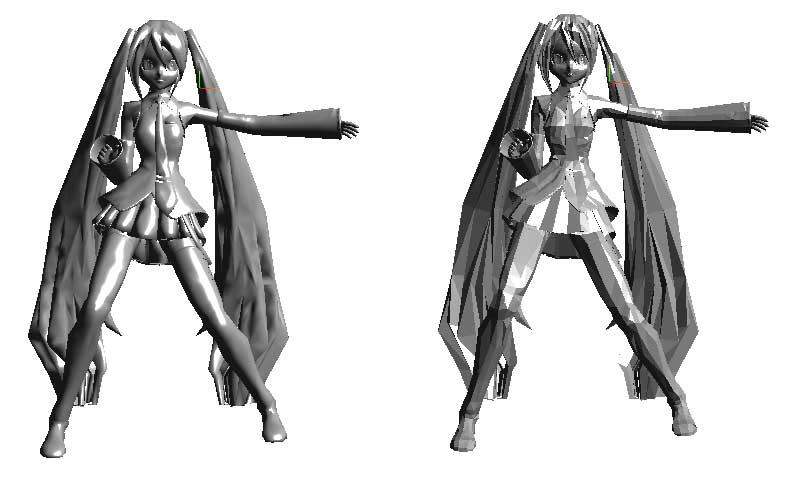图三 (左边为未加shading，右边加了shading)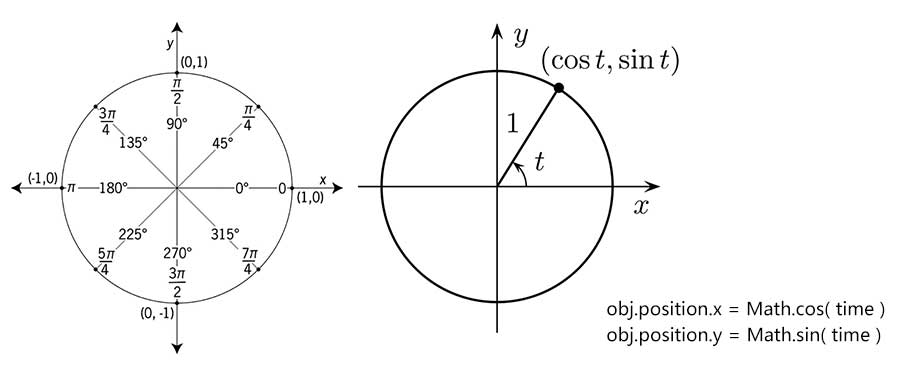图四 (圆周运动计算)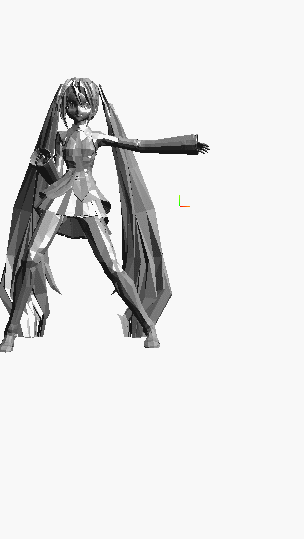图五 (圆周运动测试结果)

``````var clock = new THREE.Clock(); //时间跟踪
//圆周运动
var time = clock.getElapsedTime() * 1;
loadMesh.position.x = Math.cos( time ) * 10;
loadMesh.position.y = Math.sin( time ) * 10;
``````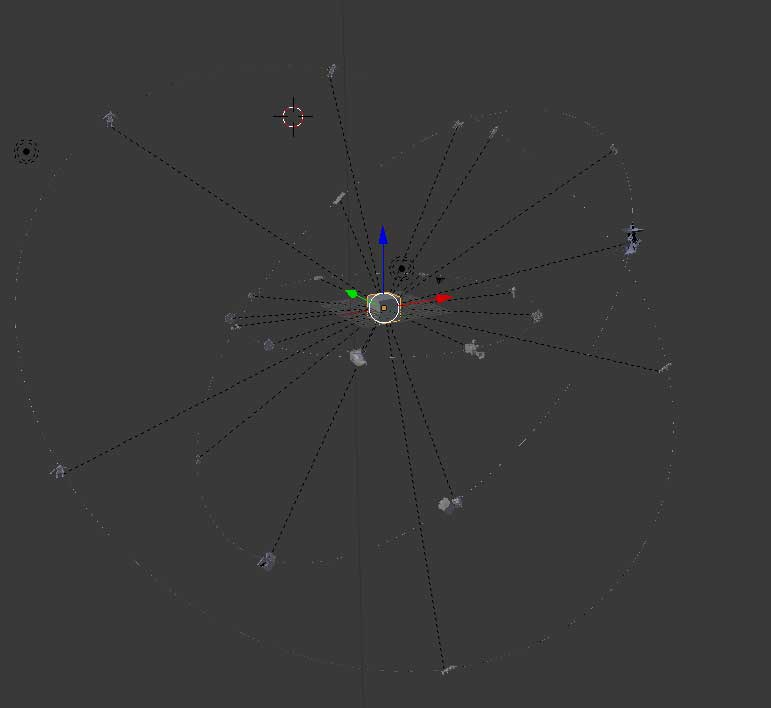图六 (球体模型)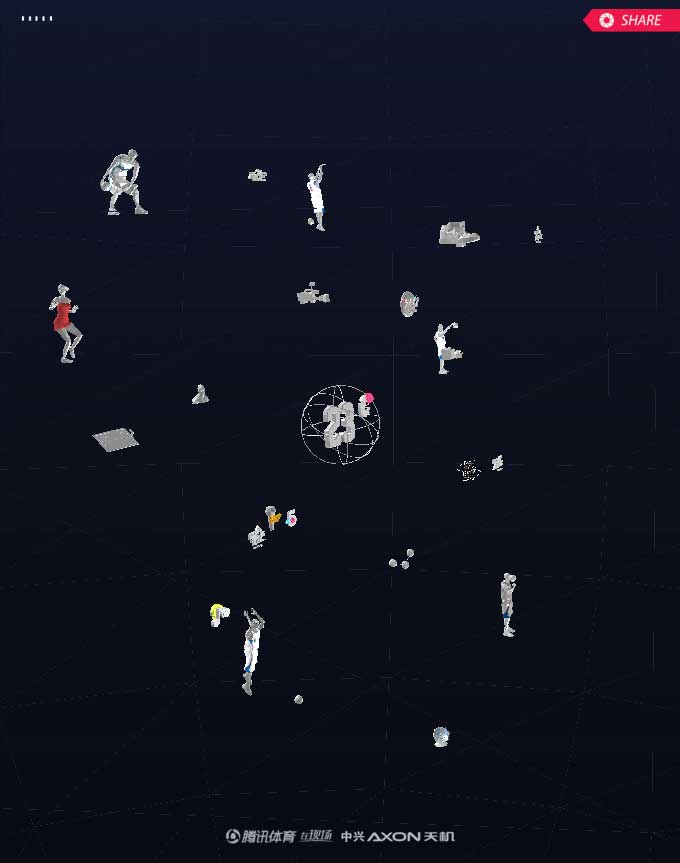图七 (页面中渲染效果)

``````mouse.x = ( e.clientX / window.innerWidth ) * 2 - 1; //鼠标的x到屏幕y轴的距离与屏幕宽的一半的比值 绝对值不超过1
mouse.y = - ( e.clientY / window.innerHeight ) * 2 + 1; //鼠标的y到屏幕x轴的距离与屏幕宽的一半的比值 绝对值不超过1

//新建一个三维变换半单位向量 假设z方向就是0.5,这样左右移的时候，还会有前后移的效果
//屏幕和场景转换工具根据照相机，把这个向量从屏幕转化为场景中的向量
var vector = new THREE.Vector3( mouse.x, mouse.y, 0.5 ).unproject( camera );

//变换过后的向量vector减去相机的位置向量后标准化
var raycaster = new THREE.Raycaster( camera.position, vector.sub( camera.position ).normalize() );

//新建一条从相机的位置到vector向量的一道光线
var intersects = raycaster.intersectObjects( objects );

if ( intersects.length > 0 ) {

//把选中的对象放到全局变量SELECTED中
SELECTED = intersects[ 0 ].object;
}
``````

（1）改变选中物体的x,y,z值

经过反复的测试发现改变x,y,z值，模型会在空间中乱窜，把握不好位置。于是就思考其它的方案。

（2）移动相机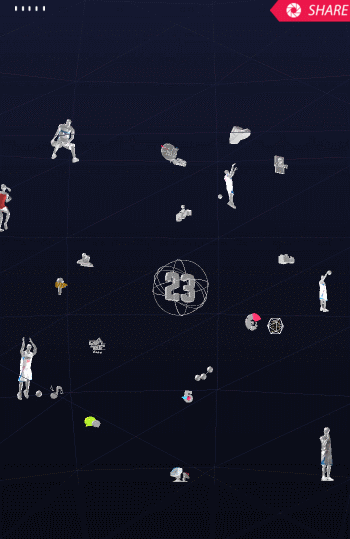图八 (相机移动)

``````TweenMax.to(camera.position, 1, {
x: x,
y: y,
z: z,
ease:Expo.easeInOut,
onComplete: function (){}
})
``````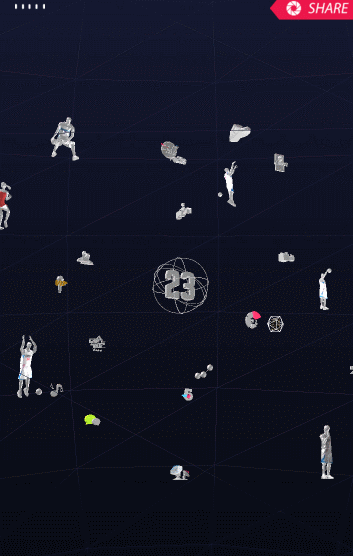图九 (相机平滑移动)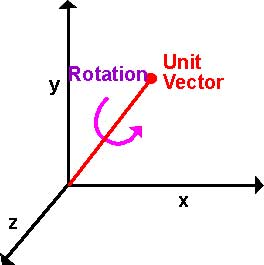图十

``````function rotateMatrix(rotateStart, rotateEnd){
var axis = new THREE.Vector3(),
quaternion = new THREE.Quaternion();

//得到开始和结束向量间的夹角
var angle = Math.acos(rotateStart.dot(rotateEnd) / rotateStart.length() / rotateEnd.length());

if (angle){  //如果夹角等于0， 说明物体没有旋转
axis.crossVectors(rotateStart, rotateEnd).normalize();  //rotateStart,rotateEnd向量乘积 标准化 得到旋转轴
angle *= _that.rotationSpeed; //rotationSpeed旋转系数 得到旋转弧度
quaternion.setFromAxisAngle(axis, angle);  //从一个旋转轴和旋转弧度得到四元组， 如果要让物体相反方向旋转 设置angle为负
}
return quaternion; //返回一个旋转的四元数
}

this.handleRotation = function(object){
_that.rotateEndPoint = _that.projectOnTrackball(_that.deltaX, _that.deltaY);
var rotateQuaternion = rotateMatrix(_that.rotateStartPoint, _that.rotateEndPoint);
var curQuaternion = object.quaternion;
curQuaternion.multiplyQuaternions(rotateQuaternion, curQuaternion); //设置四元组 a x b
curQuaternion.normalize();
object.setRotationFromQuaternion(curQuaternion);  //方法通过规范化的旋转四元数直接应用旋转  参数必须normalize()
};
``````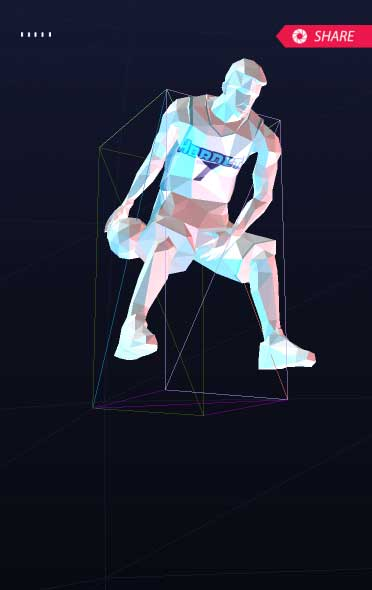图十一 (外层包裹框)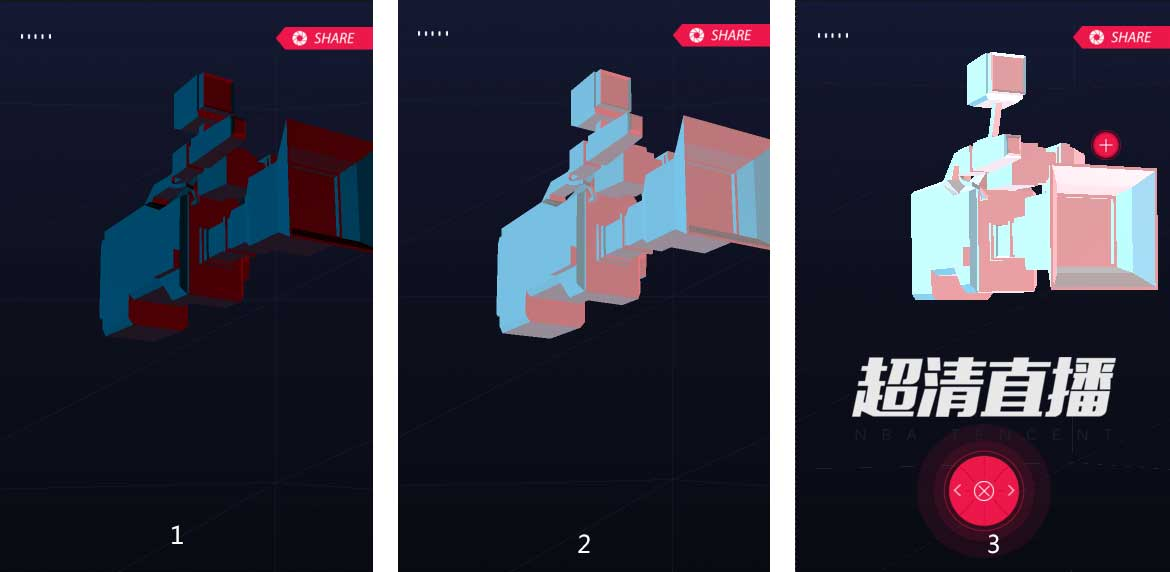图十二 (灯光调整过程)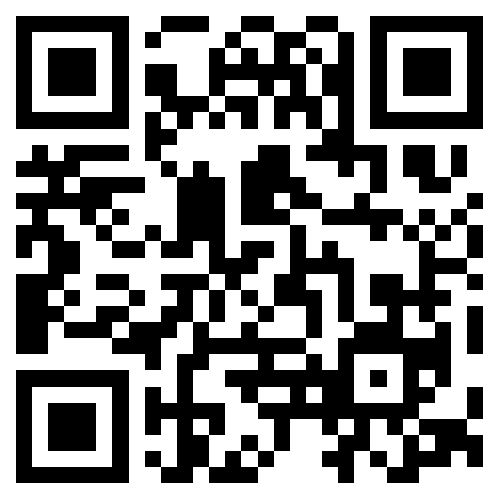扫码体验

《Three.Js Essentials》、《threejs-cookbook》、《Learning Three.js》